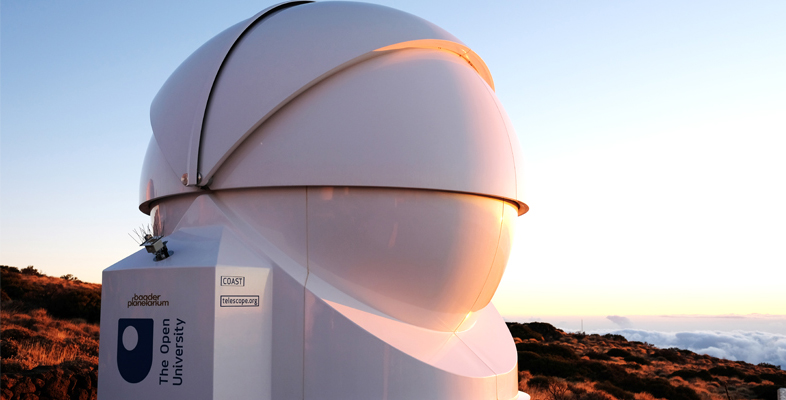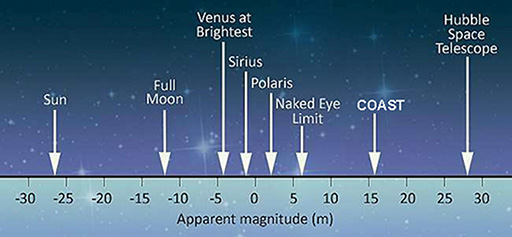Science, Maths & Technology

### Become an OU studentAstronomy with an online telescope

Start this free course now. Just create an account and sign in. Enrol and complete the course for a free statement of participation or digital badge if available.

# 1.2 Exploring the magnitude scale

To explore the magnitude scale in more detail, let’s take a look at where some familiar objects appear on the scale.Figure 2

In Figure 2, the magnitude scale has been extended to include objects far brighter than the stars – including Venus, the Moon and the Sun. Being extremely bright, these have very large negative magnitude numbers.

In order to cover such a wide range of brightness, the magnitude scale works on a logarithmic principle. This is a mathematical term meaning that each step along the scale multiplies the brightness by a certain amount, rather than simply adding a fixed amount. Specifically, a change of five magnitudes represents an increase or decrease of 100 times in the brightness – so that for example, a magnitude +1 star is one hundred times brighter than a magnitude +6 star.

Breaking this down into individual steps along the scale a change of one magnitude is equal to an increase or decrease of approximately 2.5 times in brightness.

[Many other scales work on this logarithmic principle in order to cover a wide range – the Richter scale for earthquakes and the decibel scale for intensity of sound are two other examples of logarithmic scales. In both cases, each step along the scale represents a multiplication in the value being measured.]

## Activity 2 Caculating the difference in brightness of stars

Timing: Allow approximately 5 minutes

Two stars have magnitudes of +2.0 and +4.5 – a difference of 2.5 magnitudes.

How many times brighter is the magnitude +2.0 star than the magnitude +4.5 star ?